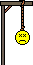# Stuck on a antiderivative

## Homework Statement

Find $$\int \frac{x^{2}+3x+7}{\sqrt{x}}dx$$

## The Attempt at a Solution

I am reviewing for a test on tuesday. I can't see a good algebraic method to solve this using the fact that $$\int f(g(x))g'(x)dx=F(g(x))+C$$ so I am starting to think it requires a u-substitution which I am trying to get better at right now before the test. Can someone point me in the right direction here? Thankyou very much, I appreciate any help!